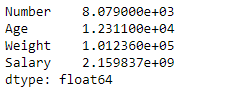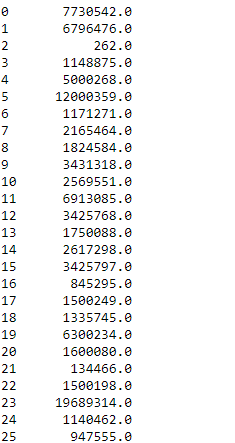Related Articles
Python | Pandas dataframe.sum()
• Difficulty Level : Basic
• Last Updated : 22 Oct, 2019

Python is a great language for doing data analysis, primarily because of the fantastic ecosystem of data-centric python packages. Pandas is one of those packages and makes importing and analyzing data much easier.

Pandas` dataframe.sum()` function return the sum of the values for the requested axis. If the input is index axis then it adds all the values in a column and repeats the same for all the columns and returns a series containing the sum of all the values in each column. It also provides support to skip the missing values in the dataframe while calculating the sum in the dataframe.

Syntax: DataFrame.sum(axis=None, skipna=None, level=None, numeric_only=None, min_count=0, **kwargs)

Parameters :
axis : {index (0), columns (1)}
skipna : Exclude NA/null values when computing the result.
level : If the axis is a MultiIndex (hierarchical), count along a particular level, collapsing into a Series
numeric_only : Include only float, int, boolean columns. If None, will attempt to use everything, then use only numeric data. Not implemented for Series.
min_count : The required number of valid values to perform the operation. If fewer than min_count non-NA values are present the result will be NA.

Returns : sum : Series or DataFrame (if level specified)

For link to the CSV file used in the code, click here

Example #1: Use `sum()` function to find the sum of all the values over the index axis.

 `# importing pandas as pd``import` `pandas as pd`` ` `# Creating the dataframe ``df ``=` `pd.read_csv(``"nba.csv"``)`` ` `# Print the dataframe``df`Now find the sum of all values along the index axis. We are going to skip the `NaN` values in the calculation of the sum.

 `# finding sum over index axis``# By default the axis is set to 0``df.``sum``(axis ``=` `0``, skipna ``=` `True``)`

Output :Example #2: Use `sum()` function to find the sum of all the values over the column axis.

Now we will find the sum along the column axis. We are going to set skipna to be true. If we do not skip the `NaN` values then it will result in `NaN` values.

 `# importing pandas as pd``import` `pandas as pd`` ` `# Creating the dataframe ``df ``=` `pd.read_csv(``"nba.csv"``)`` ` `# sum over the column axis.``df.``sum``(axis ``=` `1``, skipna ``=` `True``)`

Output :Attention geek! Strengthen your foundations with the Python Programming Foundation Course and learn the basics.

To begin with, your interview preparations Enhance your Data Structures concepts with the Python DS Course.

My Personal Notes arrow_drop_up